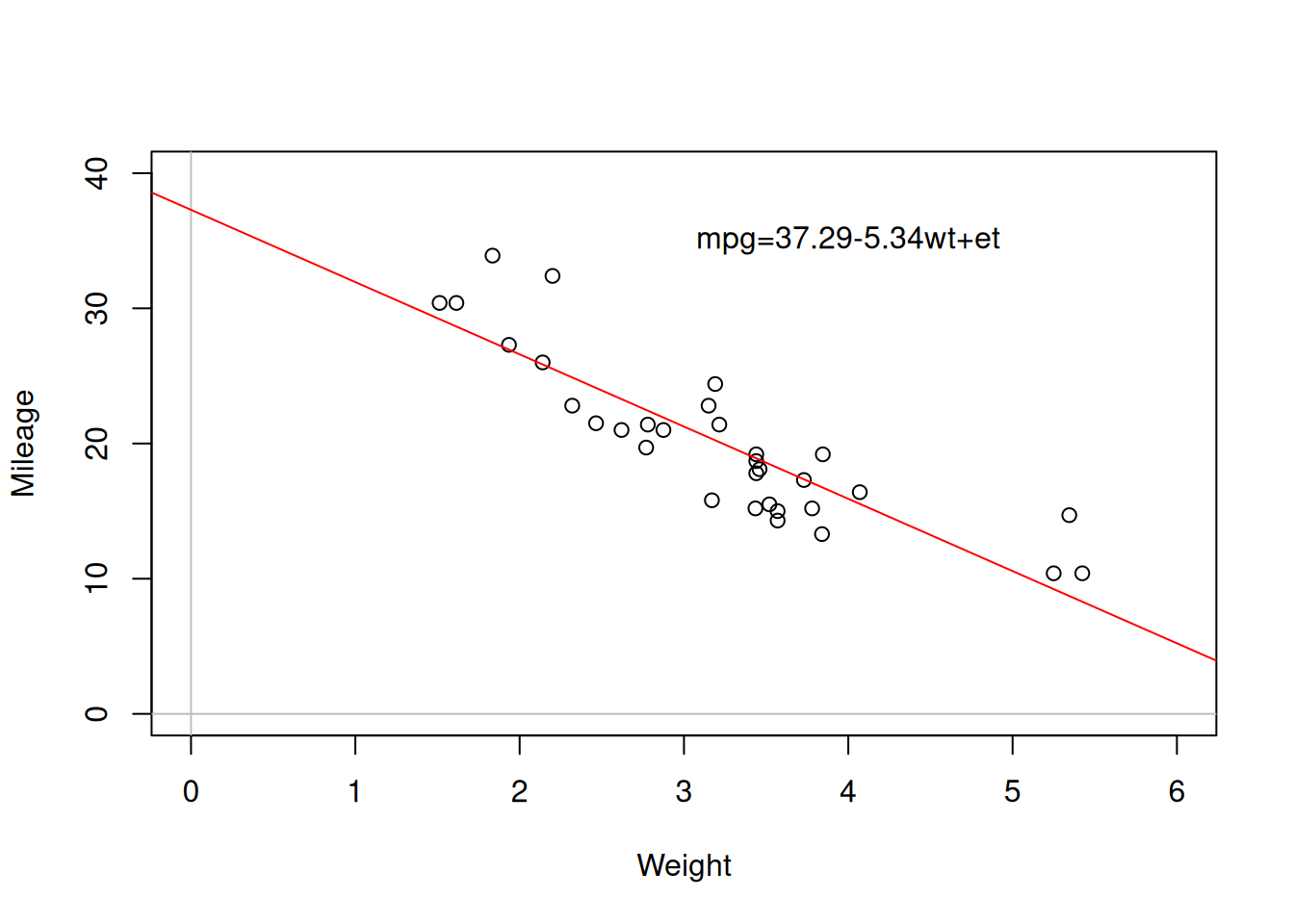This book is in Open Review. I want your feedback to make the book better for you and other readers. To add your annotation, select some text and then click the on the pop-up menu. To see the annotations of others, click the button in the upper right hand corner of the page

# Chapter 10 Simple Linear Regression

When we want to analyse some relations between variables, we can do graphical and correlations analysis. But this will not provide us sufficient information about what happens with the response variable with the change of explanatory variable. So it makes sense to consider the possible relations between variables, and the basis for this is Simple Linear Regression, which can be represented in the form: $\begin{equation} y_j = \beta_0 + \beta_1 x_j + \epsilon_j , \tag{10.1} \end{equation}$ where $$\beta_0$$ is the intercept (constant term), $$\beta_1$$ is the coefficient for the slope parameter and $$\epsilon_j$$ is the error term. The regression model is a basic statistical model that captures the relation between an explanatory variable $$x_j$$ and the response variable $$y_j$$. The parameters of the models are typically denoted as $$\beta_0$$ and $$\beta_1$$ in econometrics literature, but we use $$\beta_0$$ and $$\beta_1$$ because we will use $$\beta$$ for other purposes later in this textbook.

In order to better understand what simple linear regression implies, consider the scatterplot (we discussed it earlier in Section 5.2) shown in Figure 10.1.

slmMPGWt <- lm(mpg~wt,mtcarsData)
plot(mtcarsData$wt, mtcarsData$mpg,
xlab="Weight", ylab="Mileage",
xlim=c(0,6), ylim=c(0,40))
abline(h=0, col="grey")
abline(v=0, col="grey")
abline(slmMPGWt,col="red")
text(4,35,paste0(c("mpg=",round(coef(slmMPGWt),2),"wt+et"),collapse=""))Figure 10.1: Scatterplot diagram between weight and mileage.

The line drawn on the plot is the regression line, parameters of which were estimated based on the available data. In this case the intercept $${b}_0$$=37.29, meaning that this is where the red line crosses the y-axis, while the parameter of slope $${b}_1$$=-5.34 shows how fast the values change (how steep the line is). Note that we use $$b_0$$ and $$b_1$$ for the parameters that were estimated on a sample of data. If we had all the data in the universe (population) and estimated the correct model on it, we would use $$\beta_0$$ and $$\beta_1$$. In simple linear regression, the red line will always go through the cloud of points, showing the averaged out tendencies. The one that we observe above can be summarise as “with the increase of weight, on average the mileage of cars goes down”. Note that we might find some specific points, where the increase of weight would not decrease mileage (e.g. the two furthest points to the left show this), but this can be considered as a random fluctuation, so overall, the average tendency is as described above.